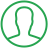# Java Random Number Between 1 And 100

Last Updated : Oct 2, 2023In this article we will show you the solution of java random number between 1 and 100, the popular programming language Java gives programmers a complete set of tools to build adaptable programmes.

Applications ranging from games to statistical models frequently call for the generation of random numbers.

Through its built-in library, java.util.Random, Java provides a trustworthy method for producing random integers.

With the use of this library, programmers may quickly create random numbers that fall within a given range.

We can make sure that impartial and unpredictable random numbers are generated by utilising Java's Random class and its methods.

## Step By Step Guide On Java Random Number Between 1 And 100 :-

```import java.util.Random;
public class RandomNumberGenerator {
public static void main(String[] args) {
Random random = new Random();
int randomNumber = random.nextInt(100) + 1;
System.out.println("Random Number between 1 and 100: " + randomNumber);
boolean isEven = randomNumber % 2 == 0;
int square = randomNumber * randomNumber;
int anotherRandomNumber = random.nextInt(100) + 1;
boolean isGreaterThan = randomNumber > anotherRandomNumber;
System.out.println("Is the random number even? " + isEven);
System.out.println("Square of the random number: " + square);
System.out.println("Another random number: " + anotherRandomNumber);
System.out.println("Is the random number greater than the another random number? " + isGreaterThan);
}
}```
1. You can see how we construct the Java code to print a random number between 1 and 100 in this section.
2. We begin by importing the java.util.Random class, which offers ways to produce random integers.
3. The RandomNumberGenerator class has the main function, which serves as the program's starting point.
4. Using the new Random() syntax, we generate a random instance of the Random class inside the main method.
5. This enables us to employ the random number-generating techniques that the Random class offers.
6. We generate a number at random between 1 and 100 by calling the nextInt(100) + 1 function.
7. A number among 0 and 100 is generated at randomly by the nextInt(100) method.
8. The range is changed to 1 (inclusive) and 101 (exclusive) by adding 1 to the result, essentially producing random numbers between 1 and 100.
9. The int-type randomNumber variable contains the generated random number.
10. Using System.out.println(), we output the produced random number along with the necessary message.
11. With the created random number, we carry out more procedures.
12. By utilising the modulo operator (%), we first determine whether the random integer is even.
13. The isEven boolean variable is set to true if the random number divided by two leaves no remainder (randomNumber% 2 == 0), indicating that the value is even.
14. If not, it is unusual, and we set isEven to false.
15. By multiplying the random number by itself, we can find its square, and we can then store the answer in an int square variable.
16. Using the nextInt(100) + 1 method call, we produce a new random number between 1 and 100.
17. The process is similar to step 4, and the produced random number is saved in the int-type variable called anotherRandomNumber.
18. Using the greater than (>) operator, we compare randomNumber and anotherRandomNumber.
19. The isGreaterThan boolean variable is set to true if randomNumber is greater than anotherRandomNumber; otherwise, it is set to false.
20. We also display additional information about the generated random numbers, such as whether or not it is even (isEven), its square (square), the value of another random number (anotherRandomNumber), and whether it is greater than another random number (isGreaterThan).

## Conclusion :-

As a result, we were able to understand the idea of a java random number between 1 and 100.

We also discovered how simple it is to get unbiased and unpredictable random numbers within a given range by making an instance of the Random class and using the nextInt() method.

I hope this article on java random number between 1 and 100 helps you and the steps and method mentioned above are easy to follow and implement.

##About Anjali

Experienced Computer Programmer with a broad range of experience in technology. Strengths in application development and Object Oriented architecture design, front end programming, usability and multimedia technology. Expert in coding languages such as C, C++ Java, JavaScript, PHP and more.